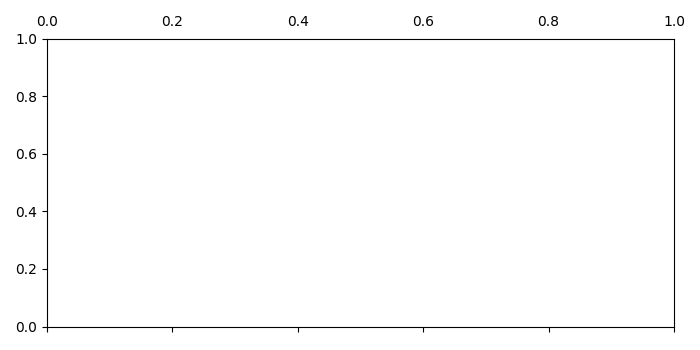# How to show tick labels on top of a matplotlib plot?

To show tick labels on top of a matplotlib plot, we can use the set_tick_params() method with labeltop=True.

## Steps

• Set the figure size and adjust the padding between and around the subplots.
• Create a figure and a set of subplots.
• Show the tick labels at the top of the plot. Use set_tick_parama() with labeltop=True.
• Hide the tick labels of the bottom axis of plot. Use set_tick_parama() with labeltop=False.
• To display the figure, use show() method.

## Example

from matplotlib import pyplot as plt

# Set the figure size
plt.rcParams["figure.figsize"] = [7.00, 3.50]
plt.rcParams["figure.autolayout"] = True

# Create subplots
fig, ax = plt.subplots(1, 1)

# Show the tick labels
ax.xaxis.set_tick_params(labeltop=True)

# Hide the tick labels
ax.xaxis.set_tick_params(labelbottom=False)

plt.show()

## Output

It will produce the following output